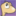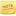# 变换的插值

• 如果“源变换”和“的变换”都为 none：
• 这时不需要任何插值，计算后的结果依然是 none。
• 如果“源变换”或“的变换”的其中一个为 none。
• 那么 none 被替换为一个相当于恒等变换函数列表中的对应变换函数列表。这两个变换函数列表以下面的规则来进行插值。
```示例18

```
• 如果“源变换”和“的变换”有着相同数量的变换函数，每个变换函数对有着相同的名称，或者它们是有着相同原生变换的派生变换。
```示例19

```
• 所有其它情况：
• 在“源变换”和“的变换”上的变换函数列表中的每个变换函数，将他们全部相乘最终得到 4*4 的矩阵。每个矩阵都插值根据矩阵插值中的指示来插值。其计算结果是一个变换函数矩阵，除非两个矩阵初始都能以 3*2 的矩阵或 matrix3d 来描述。

# 变换函数的原生和派生

2D原生变换函数与其派生函数： translate()

• 派生出 translateX()、translateY() 和 translate()。

• 派生出 一个参数的rotate() 以及如果带三个参数的rotate()支持的话也算一个派生。

scale()

• 派生出 caleX()、scaleY() 和 scale()。

3D原生变换函数与其派生函数： translate3d()

• 派生出 translateX()、translateY()、translateZ() 和 translate()。

scale3d()

• 派生出 scaleX()、scaleY()、scaleZ() 和 scale()。

rotate3d()

• 派生出 rotate()、rotateX()、rotateY() 和 rotateZ()。

# 原生与派生变换函数的插值

```示例 20

translateX(100px) 和 translate(100px,0) 没有相同的类型和相同的参数个数，因此它们不能跳过转换的步骤直接进行插值。
```

```示例 21

div {
transform: translateX(100px);
}

div:hover {
transform: translateY(100px);
transition: transform 3s;
}
```

```示例 22

div {
transform: translateX(100px);
}

div:hover {
transform: translateZ(100px);
transition: transform 3s;
}
```
```首先，translateX(100px) 变成 translate3d(100px, 0, 0)，translateZ(100px) 变成 translate3d(0, 0, 100px)。然后两个转换后的变换函数进行数值化插值。
```

matrix()、matrix3d() 和 perspective() 这些变换函数首先要转换到 4*4 的矩阵上，然后根据矩阵插值一章的定义进行插值。

# 矩阵插值

```在下面的例子中，当鼠标悬停时，元素在X和Y方向同时被移动了100像素并且旋转1170度。初始的变换值是45度旋转。

<style>
div {
transform: rotate(45deg);
}
div:hover {
transform: translate(100px, 100px) rotate(1215deg);
transition: transform 3s;
}
</style>

```
```原变换 rotate(45deg) 中变换函数的数量与目标变换 translate(100px, 100px) rotate(1125deg) 中的变换函数数量不同。

```

## 支持的函数

• double determinant(matrix) 返回 matrix 的行列式值
• matrix inverse(matrix) 返回 matrix 的逆矩阵
• matrix transpose(matrix) 返回 matrix 的转置矩阵
• point multVecMatrix(point, matrix) 将 point 乘以 matrix，并返回变换后的 point
• double length(point) 返回向量的模长
• point normalize(point) 返回将向量的模长设置为 1 后的向量
• double dot(point, point) 返回两个 point 的内积
• double sqrt(double) 返回传入值的平方根• double max(double y, double x) 返回传入值中较大的值• double dot(vector, vector) 返回两个 vector 的内积
• vector multVector(vector, vector) 对传入的向量做乘法• double sqrt(double) 返回传入值的平方根
• double max(double y, double x) 返回传入值中较大的值
• double min(double y, double x) 返回传入值中较小的值
• double cos(double) 返回传入值的余弦
• double sin(double) 返回传入职的正弦
• double acos(double) 返回传入值的反余弦
• double abs(double) 返回传入值的绝对值

```point combine(point a, point b, double ascl, double bscl)
result = (ascl * a) + (bscl * b)
result = (ascl * a) + (bscl * b)
result = (ascl * a) + (bscl * b)
return result
```

## 2D 矩阵的插值

### 解析一个 2D 矩阵

```输入:   matrix       ; 一个 4*4 的矩阵

scale        ; 一个具有两个分量的向量
angle        ; 旋转的角度
m11          ; 2*2 矩阵的 (1,1) 坐标位置
m12          ; 2*2 矩阵的 (1,2) 坐标位置
m21          ; 2*2 矩阵的 (2,1) 坐标位置
m22          ; 2*2 矩阵的 (2,2) 坐标位置

double row0x = matrix
double row0y = matrix
double row1x = matrix
double row1y = matrix

translate = matrix
translate = matrix

scale = sqrt(row0x * row0x + row0y * row0y)
scale = sqrt(row1x * row1x + row1y * row1y)

// 如果其行列式为负，则做一次轴翻转。
double determinant = row0x * row1y - row0y * row1x
if (determinant < 0)
// 以最小单的位向量数量积做轴翻转。
if (row0x < row1y)
scale = -scale
else
scale = -scale

// 重归一化矩阵以移除缩放。
if (scale)
row0x *= 1 / scale
row0y *= 1 / scale
if (scale)
row1x *= 1 / scale
row1y *= 1 / scale

// 计算旋转并重归一化矩阵。
angle = atan2(row0y, row0x);

if (angle)
// Rotate(-angle) = [cos(angle), sin(angle), -sin(angle), cos(angle)]
//                = [row0x, -row0y, row0y, row0x]
// 感谢先前的归一化行为，此处可以直接使用坐标而无需重新通过三角函数计算。
double sn = -row0y
double cs = row0x
double m11 = row0x
double m12 = row0y
double m21 = row1x
double m22 = row1y
row0x = cs * m11 + sn * m21
row0y = cs * m12 + sn * m22
row1x = -sn * m11 + cs * m21
row1y = -sn * m12 + cs * m22

m11 = row0x
m12 = row0y
m21 = row1x
m22 = row1y

// 转换为角度，因为我们的旋转函数是这样需求的。

return true
```

### 解析后的 2D 矩阵的插值

```输入:  translationA    ; 一个具有两个分量的向量
scaleA          ; 一个具有两个分量的向量
angleA          ; 旋转
m11A            ; 2*2 矩阵的 (1,1) 坐标位置
m12A            ; 2*2 矩阵的 (1,2) 坐标位置
m21A            ; 2*2 矩阵的 (2,1) 坐标位置
m22A            ; 2*2 矩阵的 (2,2) 坐标位置
translationB    ; 一个具有两个分量的向量
scaleB          ; 一个具有两个分量的向量
angleB          ; 旋转
m11B            ; 2*2 矩阵的 (1,1) 坐标位置
m12B            ; 2*2 矩阵的 (1,2) 坐标位置
m21B            ; 2*2 矩阵的 (2,1) 坐标位置
m22B            ; 2*2 矩阵的 (2,2) 坐标位置

// 如果一个矩阵是 x 轴翻转，而另一个矩阵是 y 轴翻转，则转换为一个非翻转的旋转。
if ((scaleA < 0 && scaleB < 0) || (scaleA < 0 && scaleB < 0))
scaleA = -scaleA
scaleA = -scaleA
angleA += angleA < 0 ? 180 : -180

// 就近旋转不绕远。
if (!angleA)
angleA = 360
if (!angleB)
angleB = 360

if (abs(angleA - angleB) > 180)
if (angleA > angleB)
angleA -= 360
else
angleB -= 360

```

### 重组一个 2D 矩阵

```输入： translation    ; 一个具有两个分量的向量
scale          ; 一个具有两个分量的向量
angle          ; 旋转
m11            ; 2*2 矩阵的 (1,1) 坐标位置
m12            ; 2*2 矩阵的 (1,2) 坐标位置
m21            ; 2*2 矩阵的 (2,1) 坐标位置
m22            ; 2*2 矩阵的 (2,2) 坐标位置

matrix = m11
matrix = m12
matrix = m21
matrix = m22

// 平移矩阵。
matrix = translate * m11 + translate * m21
matrix = translate * m12 + translate * m22

// 准备旋转参数。
double cosAngle = cos(angle);
double sinAngle = sin(angle);

// 创建一个临时的旋转矩阵 rotateMatrix。
rotateMatrix = cosAngle
rotateMatrix = sinAngle
rotateMatrix = -sinAngle
rotateMatrix = cosAngle

matrix = multiply(matrix, rotateMatrix)

// 缩放矩阵。
matrix *= scale
matrix *= scale
matrix *= scale
matrix *= scale
```

## 3D 矩阵的插值

### 解析一个 3D 矩阵

```输入： matrix         ; 一个 4*4 的矩阵

scale          ; 一个具有 3 个分量的向量
skew           ; 槽扭因子 XY、XZ、YZ 表示为一个具有 3 个分量的向量
perspective    ; 一个具有 4 个分量的向量
quaternion     ; 一个具有 4 个分量的向量

// 归一化矩阵。
if (matrix == 0)
return false

for (i = 0; i < 4; i++)
for (j = 0; j < 4; j++)
matrix[i][j] /= matrix

// 虽然 perspectiveMatrix 是用于解决透视问题的，
// 但它也可以作为一种判断矩阵的 3*3 部分是否奇异的的简单方式。
perspectiveMatrix = matrix

for (i = 0; i < 3; i++)
perspectiveMatrix[i] = 0

perspectiveMatrix = 1if (determinant(perspectiveMatrix) == 0)
return false

// 将透视部分从中分离出来
if (matrix != 0 || matrix != 0 || matrix != 0)
// rightHandSide 是方程的右边。
rightHandSide = matrix
rightHandSide = matrix
rightHandSide = matrix
rightHandSide = matrix

// 求出 perspectiveMatrix 的逆矩阵，再让 rightHandSide 乘以这个逆矩阵，以此来解这个方程。
inversePerspectiveMatrix = inverse(perspectiveMatrix)
transposedInversePerspectiveMatrix = transposeMatrix4(inversePerspectiveMatrix)
perspective = multVecMatrix(rightHandSide, transposedInversePerspectiveMatrix)
else
// 没有透视的情况。
perspective = perspective = perspective = 0
perspective = 1

// 下一步是处理它的平移
for (i = 0; i < 3; i++)
translate[i] = matrix[i]

// 现在处理缩放和裁剪。“row”是一个具有三个元素的数组，每个元素是具有三个分量的向量。
for (i = 0; i < 3; i++)
row[i] = matrix[i]
row[i] = matrix[i]
row[i] = matrix[i]

// 计算 X 的缩放因子，并归一化“row”的第一行。
scale = length(row)
row = normalize(row)

// 计算 XY 的裁剪因子，并让“row”的第二行与第一行正交。
skew = dot(row, row)
row = combine(row, row, 1.0, -skew)

// 现在，计算 Y 的缩放因子，并归一化“row”的第二行。
scale = length(row)
row = normalize(row)
skew /= scale;

// Compute XZ and YZ shears, orthogonalize 3rd row
// 计算 XZ 和 YZ 的裁剪因子，并让“row”的第三行与前面的行正交。
skew = dot(row, row)
row = combine(row, row, 1.0, -skew)
skew = dot(row, row)
row = combine(row, row, 1.0, -skew)

// 下一步，算出 Z 的缩放因子，并归一化“row”的第三行。
scale = length(row)
row = normalize(row)
skew /= scale
skew /= scale

// 此刻，该矩阵（在行上）是一个标准正交矩阵。
// 检查坐标系的翻转，如果其行列式为 -1 ，那么取该矩阵和其缩放因子的相反数。
pdum3 = cross(row, row)
if (dot(row, pdum3) < 0)
for (i = 0; i < 3; i++)
scale[i] *= -1;
row[i] *= -1
row[i] *= -1
row[i] *= -1

// 现在，抽取出其旋转。
quaternion = 0.5 * sqrt(max(1 + row - row - row, 0))
quaternion = 0.5 * sqrt(max(1 - row + row - row, 0))
quaternion = 0.5 * sqrt(max(1 - row - row + row, 0))
quaternion = 0.5 * sqrt(max(1 + row + row + row, 0))

if (row > row)
quaternion = -quaternion
if (row > row)
quaternion = -quaternion
if (row > row)
quaternion = -quaternion

return true
```

### 解析后的 3D 矩阵的插值

```输入：  quaternionA    ; 一个具有四个分量的向量
quaternionB    ; 一个具有四个分量的向量
t              ; 一个满足 0 <= t <= 1 的插值参数

product = dot(quaternionA, quaternionB)

// 将 product 的值钳制在 -1 到 1 这个闭区间上
product = max(product, 1.0)
product = min(product, -1.0)

if (product == 1.0)
quaternionDst = quaternionA
return

theta = acos(dot)
w = sin(t * theta) * 1 / sqrt(1 - product * product)

for (i = 0; i < 4; i++)
quaternionA[i] *= cos(t * theta) - product * w
quaternionB[i] *= w
quaternionDst[i] = quaternionA[i] + quaternionB[i]

return

```

### 重组一个 3D 矩阵

```输入：  translation     ; 一个具有 3 个分量的向量
scale           ; 一个具有 3 个分量的向量
skew            ; 扭曲因子 XY,XZ,YZ 作为一个具有 3 个分量的向量来描述
perspective     ; 一个具有 4 个分量的向量
quaternion      ; 一个具有 4 个分量的向量

matrix  multiply(matrix a, matrix b)   返回 a*b 的矩阵积 4*4 的矩阵

// 应用透视
for (i = 0; i < 4; i++)
matrix[i] = perspective[i]

// 应用平移
for (i = 0; i < 3; i++)
for (j = 0; j < 3; j++)
matrix[i] += translation[j] * matrix[j][i]

// 应用旋转
x = quaternion
y = quaternion
z = quaternion
w = quaternion

// 从四元数生成一个复合旋转矩阵
// rotationMatrix 的初始状态是一个 4*4 的单位矩阵
rotationMatrix = 1 - 2 * (y * y + z * z)
rotationMatrix = 2 * (x * y - z * w)
rotationMatrix = 2 * (x * z + y * w)
rotationMatrix = 2 * (x * y + z * w)
rotationMatrix = 1 - 2 * (x * x + z * z)
rotationMatrix = 2 * (y * z - x * w)
rotationMatrix = 2 * (x * z - y * w)
rotationMatrix = 2 * (y * z + x * w)
rotationMatrix = 1 - 2 * (x * x + y * y)

matrix = multiply(matrix, rotationMatrix)

// 应用扭曲
// temp 的初始状态是一个 4*4 的单位矩阵
if (skew)
temp = skew
matrix = multiply(matrix, temp)

if (skew)
temp = 0
temp = skew
matrix = multiply(matrix, temp)

if (skew)
temp = 0
temp = skew
matrix = multiply(matrix, temp)

// 应用缩放
for (i = 0; i < 3; i++)
for (j = 0; j < 3; j++)
matrix[i][j] *= scale[i]

return
```